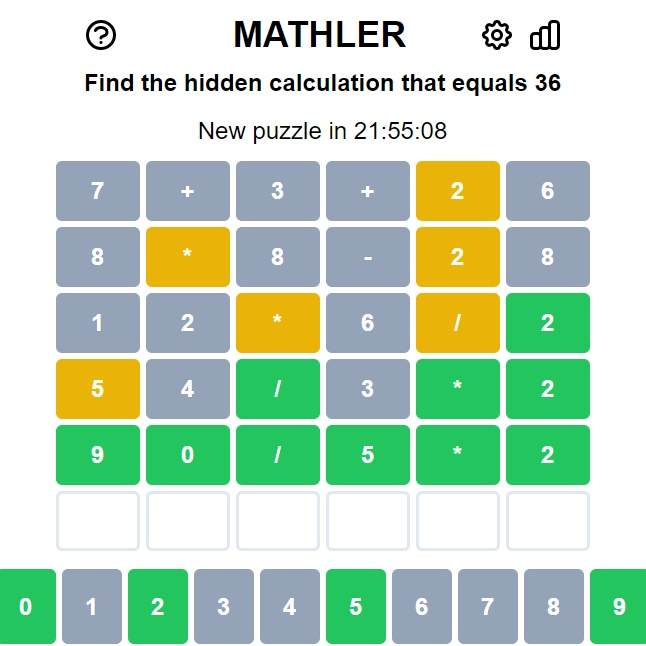Mathler
Mathler is a math game designed to appeal to math lovers from all over the world. This game is great for those who like the novelty of the idea but do not particularly enjoy the word-guessing aspect.Mathler
6 2.75
Fullscreen

# Mathler

Share

Mathler is a math game designed to appeal to math lovers from all over the world. This game is great for those who like the novelty of the idea but do not particularly enjoy the word-guessing aspect.

## How to play Mathler

In this math-based version of Wordle, players have to figure out what the "hidden calculation" is. In this case, the answer is a number, which is different from Wordle. The challenge is to figure out how to get to the number using the numbers 0 through 9 and the +, -, *, and / or symbols in the six boxes.

There are various ways to get the answer on any given day, making it harder than it sounds. For example, if the answer is 20, the solution could be 1*5+15 or 30-8-2. Figuring out which path is the right one is the hard part.

There may be repeated numbers and operations in the answer. In addition, Mathler supports commutative solutions, meaning that a+b+c is treated exactly the same as a+c+b, b+a+c, b+c+a, and c+a+b. As a result, the commutative solution will be rearranged into the exact solution automatically.

## What are the rules of Mathler?

Mathler is light on rules and heavy on simplicity, like Wordle (yes, we'll say it a lot):

• You have to guess the solution in six goes or less
• Every equation you enter must be a valid answer. So if the answer is 20, your guess can't be 50+5-2 because that doesn't equal 20. Right away, this is one of the toughest things about it.
• According to the PEDMAS rule, the equations are given in the following order: parentheses, exponentials, division, multiplication, addition, and subtraction.
• A correct entry turns green.
• A correct entry in the wrong place turns yellow.
• An incorrect entry turns gray.

Easy Mathler and Hard Mathler are the two other modes that can be chosen, with the former having five squares and one operator; the latter having eight squares and three operators. Mathler seems to get the difficulty level just right, and by using the familiar Wordle pattern, it's easy to learn. If you like word games, try our Wordle alternatives.

Comment (2)